# Nuclear Power Plants Notes | Study Mechanical Engineering SSC JE (Technical) - Mechanical Engineering

## Mechanical Engineering: Nuclear Power Plants Notes | Study Mechanical Engineering SSC JE (Technical) - Mechanical Engineering

The document Nuclear Power Plants Notes | Study Mechanical Engineering SSC JE (Technical) - Mechanical Engineering is a part of the Mechanical Engineering Course Mechanical Engineering SSC JE (Technical).
All you need of Mechanical Engineering at this link: Mechanical Engineering

NUCLEAR POWER PLANTS

• All matter is composed of unit particles called atoms. An atom consists of a relatively heavy, positively charged nucleus and a number of much lighter negatively charged, electrons orbiting around the nucleus. The nucleus consists of protons and neutrons, which together are called nucleons. Protons are positively charged, while the neutrons are electrically neutral. The atom as whole is electrically neutral, since the number of protons is equal to the number of electrons in orbit. 
• The number of protons in the nucleus is called the atomic number Z. The total number of nucleons in the nucleus is called the mass number. A nuclear symbols are written conventionally as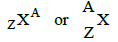Mot of the weight of an atom is concentrated in the nucleus. The radius of a nucleus is of the order of 10–16 m and that of atom is 10–11 m. The masses of the three primary atomic sub particles are :

Neutron mass. mn = 1.008665 amu = 1.674 × 10–27 kg
Proton mass, mp = 1.007277 amu = 1.673 × 10– 27 kg
Electron mass, me = 0.0005486 amu = 9.109 × 10–31 kg

The atomic mass unit, amu is a unit of a mass approximately equal to 1.66 × 10–27 kg. 

• Hydrogen (1H1) has a nucleus composed of one proton, no neutron, and one orbital electron (Z = 1, A = 1). It is the only atom that has no neutron. 
• Deuterium (1H2) has one proton and one neutron in its nucleus and one orbital electron (Z = 1, A = 2). 
• Helium (2He4) has two protons, two neutrons and two electrons (Z = 2, A = 4).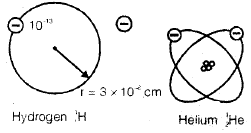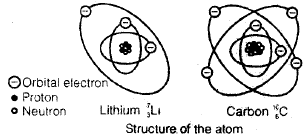Isotopes

• Atoms having same no. of proton but different no. of neutrons are known as isotopes.
Ex. U235, U238, U233, H1, H2, H3,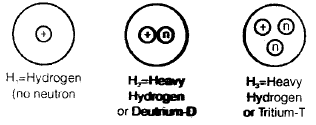Chemical and Nuclear Reactions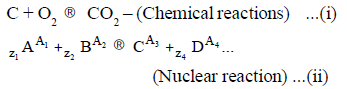Where, z1 + z2 = z3 + z

• In chemical reaction atoms as a unit takes part and electrons are exchanged. While in nuclear reactions, nuclei of atoms takes part in reaction and no. of nucleons are altered. So a new atom is formed. 
• The sum of the masses of protons and neutrons taking part is reaction is greater than that of product nucleons. This difference is called mass defect. 
• The mass defect is converted into energy by low
DE =DmC
DE = Energy
DM = Mass defect
C = Speed of light = 3 × 108 
• The energy associated with mass defect is known as binding energy as this is the amount of energy which binds the nucleon together. Binding energy is to be supplied to a nuclei to disintegrate it into its constituent nucleons.
Equivalent energy of 1 amu mass is 931 ev. 1 ev = 1. 6021 × 10– 19 J.

Radioactive Decay and Half Life 

• a-Radiation : The a-particles contains helium nuclei, containing two neutrons and two protons (2He4). Due to a-decay mass number is reduced by 4, while atomic number is reduced by 2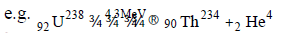•  b-Radiation : It is equivalent to emission of an electron and raises the atomic number by one, while the mass number remains the same. It is usually accompanied by the emission of neutrino (n) and g-radiation.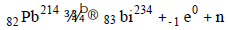• g-radiations : Gamma radiations is an electromagnetic radiation of extremely short wavelength and very high frequency. By emitting g-radiation, an excited daughter nucleus falls back is into its stable ground (lowest) energy state.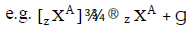It is clear that g decay does not effect either the atomic or mass number 
• Penetrating power of emissions is g > b > a. 
• Neutron emission : If a nucleus of a radioactive isotope remains in highly excited state, the emission of neutrons from the nucleus takes place. in this the mass number of the daughter isotope is reduced by unity as given by the following equation :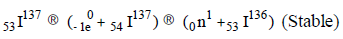• The rate of radioactive decay is a function only of the number of radioactive nuclei present at a time, provided that the number is large. It does not depend on temperature, pressure as the physical and chemical states of matter.
So; if N be the number of radioactive nuclei of one species at any time q, the rate of decay.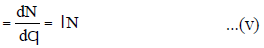l is constant of proportionality, called the decay constant, with the dimension s–1
By integrating, both sides, we get N = N0e–lq
N0 = radioactive atoms present at time q = 0
N = Number of radioactive atoms at time q
The rate of decay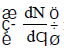is also called activity,,
A and has dimension of S–1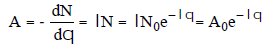The decay rate is often expressed in the form of half-life, q1/2 i.e. time during which one-half of the number of radioactive species decay.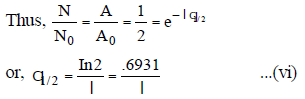Starting with N0, half of N0 decay after one half-life, one half of the remaining atoms or 1/4 of N0 decay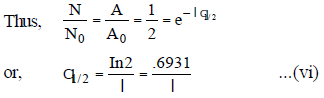Starting with N0, half of N0 decay after one half-life, one half of the remaining atoms or 1/4 of N0 decay during the second half life and so on. Half-life of some of the materials are given below:

 Metal Half life Po-214 170 µS I-137 25 Sec Carbon-14 5100 years Th-232 1.4 × 1010 years Uranium-238 4.525 × 109 years Uranium-235 7.1 × 108 year
• Unit of radioactivity is in curie (Ci) 1 Curie = 3.615 × 1010 dis/s
SI unit of Bequeral which is defined as one disintegration per second.

Nuclear Fission 

• The nuclei of atom is first bombarded with a-particle protons or neutrons. After absorbing an extra particle, the nuclei becomes unstable and nuclear fission occurs releasing energy and other products. 
• Isotopes like U-233, U-235, U-239, are bombarded with neutrons and after fission reaction energy is released in nuclear reactor. 
• Fuel burn up is the amount of energy produced in MW-days of each metric ton of fuel. Neutron Scattering In neutron scattering process, energy is transferred from one neutron to other neutron by collision. 
• A moderator is used to slow down the neutron is reactor.

PRESSURISED WATER REACTOR (PWR)

• The arrangement of pressurised water reactor in shown in figure. In its simplest form, a PWR is a light water cooled and moderated reactors. It used enriched uranium as fuel. Main component of PWR. 
• Pressuring tank 
• Electric heating coil 
• Pump 
• Reactor Core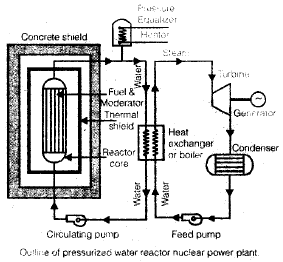• The pressurizing tank included in the circuit maintains the constant pressure in  the circuit throughout the load range. Electric heating coil in the pressurizer boils the water to form the steam which is collected in the dome as shown in figure and pressurizes the entire coolant circuit before starting reactor. To reduce the pressure, water spray is used to condense the steam.
• Operation  The water in the primary circuit gets heated by absorbing the fission energy in the reactor core and same energy is given in the heat exchanger to generate the steam.The water coming out of the heat exchanger is circulated by the pump to maintain the pressure in the circuit in the range of 100 to 130 bar.
• Precautions  The water becomes radioactive in passing through the reactor, therefore, the entire primary circuit including steam generator (heat exchanger) must be shielded to protect the operating persons. The radioactive coolant does not make the steam radioactive in the boiler. 

• The water which is used as coolant, moderator and reflector is available in plenty. 
• The reactor is compact and higher power density 
• It requires small numbers of control rods. e.g. 60 control rods in 1000 WM plant of of this type 
• The separation of secondary circuit from primary circuit provide capability to optimize the turbine cycle for the purpose of low heat rate supply. 
• It provides complete freedom to inspect and maintains the turbine, feed heaters and condenser during operation. 
• PWR allows to reduce the fuel cost extracting more energy per unit weight of fuel as PWR is ideally suited to the utilization of feed designed for higher burnups. 
• Due to high negative temperature coefficient safe and stable operation and safe regulation of the reactor is possible. 
• When more power is demanded, the reactor responds to supply the same. 

• High primary circuit pressure requires strong pressure vessel and so high capital cost. 
• Due to low pressure (60 bar), the thermodynamic efficiency of this plant is an (low as 20%). 
• The corrosion problems are more serve as the corrosion is accelerated in the presence of high pressure, high temperature etc. 
• It is necessary to shut down the reactor for feed injector which requires a couple of month time.

BOILING WATER REACTOR (BWR)

• In this type of reactor, enriched uranium is used as fuel and water is used as coolant, moderator and reflector, Unlike PWR the steam is generated in the reactor itself instead of separate steam boiler, which is shown in the figure. Bolling water reactor power plant with single circuit 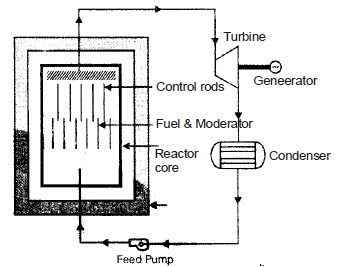• The pressure inside the reactor used is considerably smaller than PWR as water is allowed to boil inside the reactor. This reduces the cost of pressure vessel considerably. 
• This reactor does not require steam generator, pressurizers, circulating pump and connecting piping. Therefore, the cost is further reduced 
• The metal temperature remains low for given output condition. 
• A BWR is more stable than PWR and much more stable than any other type of reactor. Increases in reactivity increases the steam formations. BWR is called Self-Controlled reactors. 
• The thermal efficiency of the reactor plant (30%) is much higher than PWR (20-22%). 

• Possibility of radioactive contamination in the turbine mechanism, would these any failure of fuel elements. 
• More elaborate safety fractions which are costly. 
• Wastage of steam resulting in lowering of thermal efficiency on part load operation. 
• Boiling limits power density; only 3 to 5% by mass can be converted to steam per pass through the boiler. 
• The possibility of “Burn-out” if fuel is more in the reactor than PWR as boiling of water on the surface of the fuel is allowed.

GAS COOLED REACTOR

•  In such a type of reactors, the coolant used can be air, hydrogen, or carbon dioxide. Generally inert gases e.g. helium and carbon dioxide are used. The moderator used is graphite. This type of reactor is more safe specially in case of accidents and cooled reactors shield is much reduced as compared to the after type of reactors.
These are maimnly two types of gas cooled reactors:
• The gas cooled graphite moderator reactor (GCGM) 
• The high temperature gas cooled reactor (HTGC)
Both are graphite moderator. GCGM used natural uranium fuel while HTGC employes highly enriched uranium carbide mixed with thorium carbide and clad with graphite.

LIQUID METAL FAST BREEDER REACTOR

• Fast breeder reactors are designed to create or breed new fissile material, while producing useful electric power. Most produce fissible plutonium from uranium238. A fast neutron reaction with U-238 producing Pu-235 is shown below.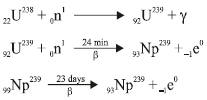When a neutron is absorbed in the fuel, it produce h neutrons, the numbers depending on the kind of feed. The conversion or breeding ratio C is given as C = h – 1 – L
Where, h = number of neutrons produced
L = number of neutrons lost of parasitic capture in reactor coolant etc. and by leakage

The maximum possible C, Cmax, will occur if L = 0, or Cmax = h – 1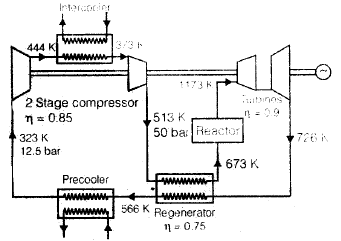A reactor with C < 1 is called a burner. A reactor with a low C is called a converted, and one with high C but less than 1.0 is called an advanced converted. When C = 1, it produce as much fissionable nuclei as it consumes. When C > 1, it breeds more nuclei than it consumes.
When C > 1, it breed more nuclei than it consumes. A reactor with C > 1 is called a breeder. G = C – 1 = h – 2 – L
Where, G = breeding gain or gain in fissionable nuclei per fissionable nucleus consumed. Similarly, maximum again.
Gmax = n – 2 

• The doubling time is the time required to produce fissionable nuclei twice the number of fissionable nuclei consumed. 
• The high concentration of fissible fuel and the absence of moderate makes the core of fast reactor smaller than a thermal reactor of the same power. With a high power density, thin fuel pins are used to minimize the temperature drop through the fuel. A coolant with excellent heat transfer properties is required to minimize the temperature drop from the fuel surface to the coolant and also it must be non-moderating. the best coolants for fast reactors are liquid metals such as sodium. Liquid metals have the additional advantage that their boiling points at atmospheric pressure are very high, so most of them can be used unpressurized in a reactor. Sodium is the most common coolant for fast reactors. However, it becomes radioactive as a result of a non-fission reaction.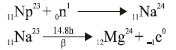where Na24 is a highly radioactive isotopic that emits 2.76 MeV. g radiation and 1.3 MeV b decays with a 14.8 h half-life to stable Mg24.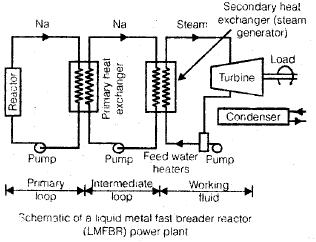• Because of this included radioactivity of liquid sodium, an intermediate loop also using Na or NaK as coolant is used between the primary radioactive coolant and the steam cycle.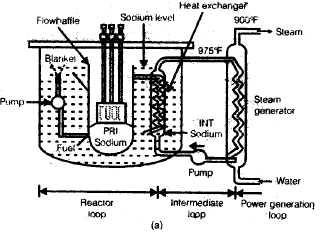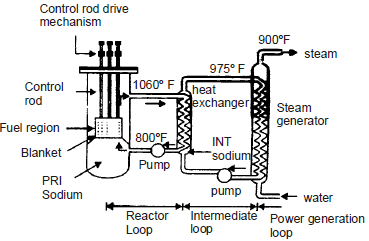• There are two primary loop designs : 
• The pool type system 
• The loop type system 
• In the pool type system the reactor core, primary pumps and intermediate heat exchangers are all placed in a large pool of liquid sodium contained in the reactor vessel. The liquid sodium is discharged from the intermediate heat exchanger to the pool. It is then pumped upward through the core and re-enters the heat exchanger. In the loop type system, the intermediate heat exchanger is located outside the reactor vessel.

HEAVY WATER REACTORS

• It uses heavy water (99.8% deuterium oxide D2O) as moderator and coolant as well as neutron reflector. The natural uranium (0.7% U235) is used as fuel.

Location of Nuclear Power Plant 

• Availability of cooling water 
• Transport facilities 
• Distance from load entre 
• Safety 
• Radiation waste disposal facility 
• Foundation requirement
The document Nuclear Power Plants Notes | Study Mechanical Engineering SSC JE (Technical) - Mechanical Engineering is a part of the Mechanical Engineering Course Mechanical Engineering SSC JE (Technical).
All you need of Mechanical Engineering at this link: Mechanical EngineeringUse Code STAYHOME200 and get INR 200 additional OFF

## Mechanical Engineering SSC JE (Technical)

6 videos|89 docs|55 tests

Track your progress, build streaks, highlight & save important lessons and more!

,

,

,

,

,

,

,

,

,

,

,

,

,

,

,

,

,

,

,

,

,

;